3 Individual geoms

3.1 Basic plot types

These geoms are the fundamental building blocks of ggplot2. They are useful in their own right, but are also used to construct more complex geoms. Most of these geoms are associated with a named plot: when that geom is used by itself in a plot, that plot has a special name.

Each of these geoms is two dimensional and requires both x and y aesthetics. All of them understand colour (or color) and size aesthetics, and the filled geoms (bar, tile and polygon) also understand fill.

• geom_area() draws an area plot, which is a line plot filled to the y-axis (filled lines). Multiple groups will be stacked on top of each other.

• geom_bar(stat = "identity") makes a bar chart. We need stat = "identity" because the default stat automatically counts values (so is essentially a 1d geom, see Section 5.4. The identity stat leaves the data unchanged. Multiple bars in the same location will be stacked on top of one another.

• geom_line() makes a line plot. The group aesthetic determines which observations are connected; see Chapter 4 for more detail. geom_line() connects points from left to right; geom_path() is similar but connects points in the order they appear in the data. Both geom_line() and geom_path() also understand the aesthetic linetype, which maps a categorical variable to solid, dotted and dashed lines.

• geom_point() produces a scatterplot. geom_point() also understands the shape aesthetic.

• geom_polygon() draws polygons, which are filled paths. Each vertex of the polygon requires a separate row in the data. It is often useful to merge a data frame of polygon coordinates with the data just prior to plotting. Section 6 illustrates this concept in more detail for map data.

• geom_rect(), geom_tile() and geom_raster() draw rectangles. geom_rect() is parameterised by the four corners of the rectangle, xmin, ymin, xmax and ymax. geom_tile() is exactly the same, but parameterised by the center of the rect and its size, x, y, width and height. geom_raster() is a fast special case of geom_tile() used when all the tiles are the same size. .

Each geom is shown in the code below. Observe the different axis ranges for the bar, area and tile plots: these geoms take up space outside the range of the data, and so push the axes out.

df <- data.frame(
x = c(3, 1, 5),
y = c(2, 4, 6),
label = c("a","b","c")
)
p <- ggplot(df, aes(x, y, label = label)) +
labs(x = NULL, y = NULL) + # Hide axis label
theme(plot.title = element_text(size = 12)) # Shrink plot title
p + geom_point() + ggtitle("point")
p + geom_text() + ggtitle("text")
p + geom_bar(stat = "identity") + ggtitle("bar")
p + geom_tile() + ggtitle("raster")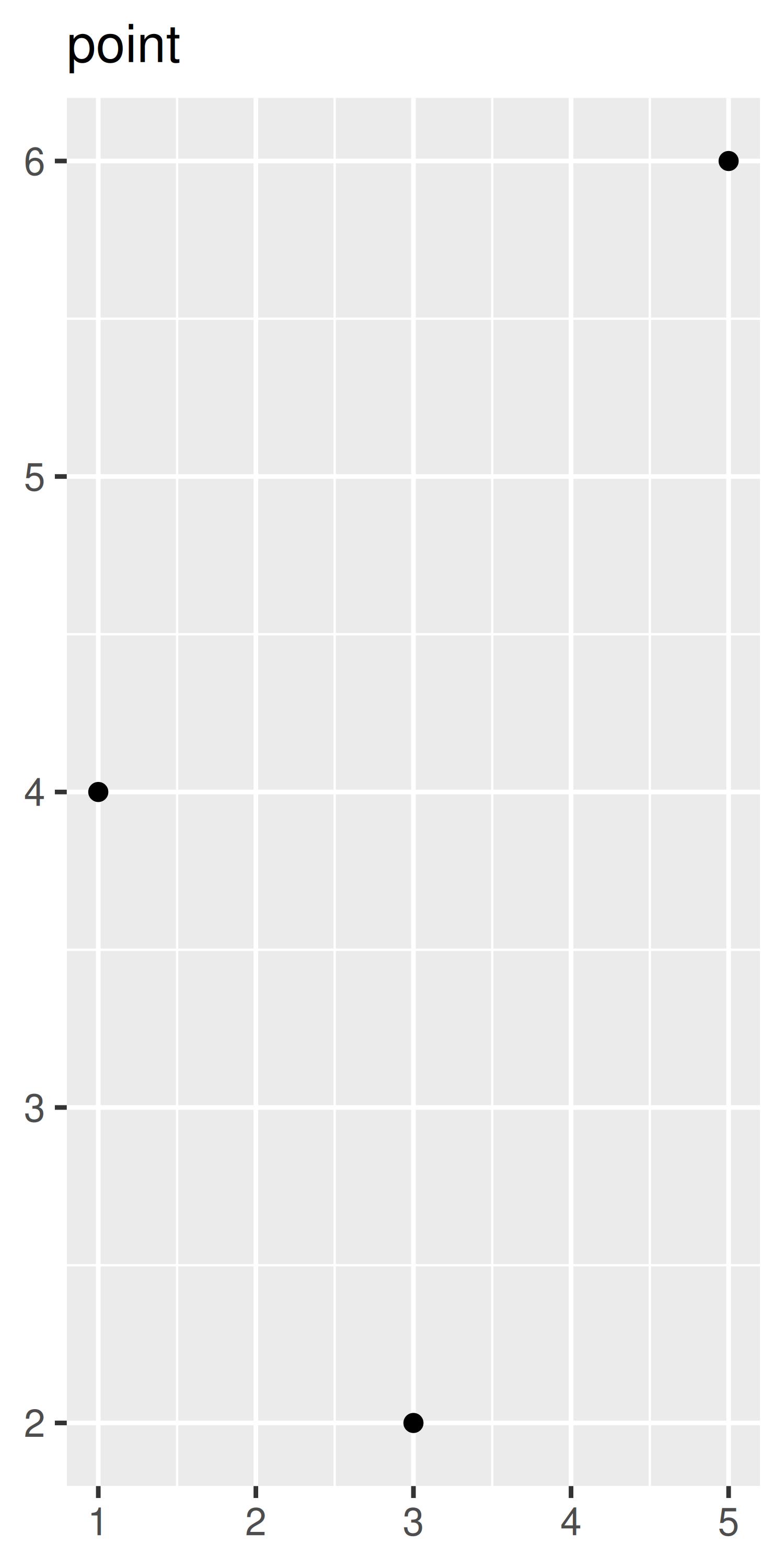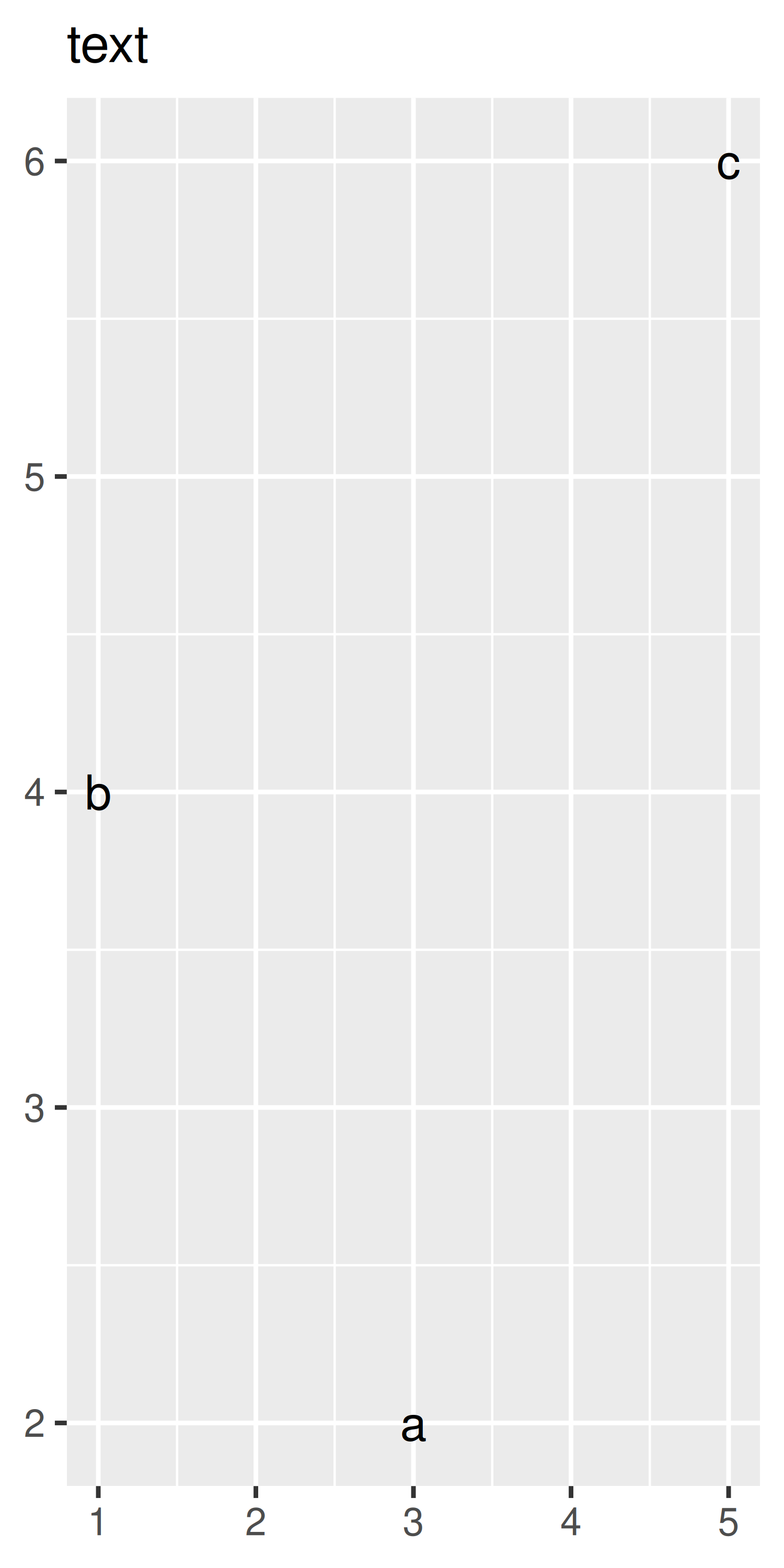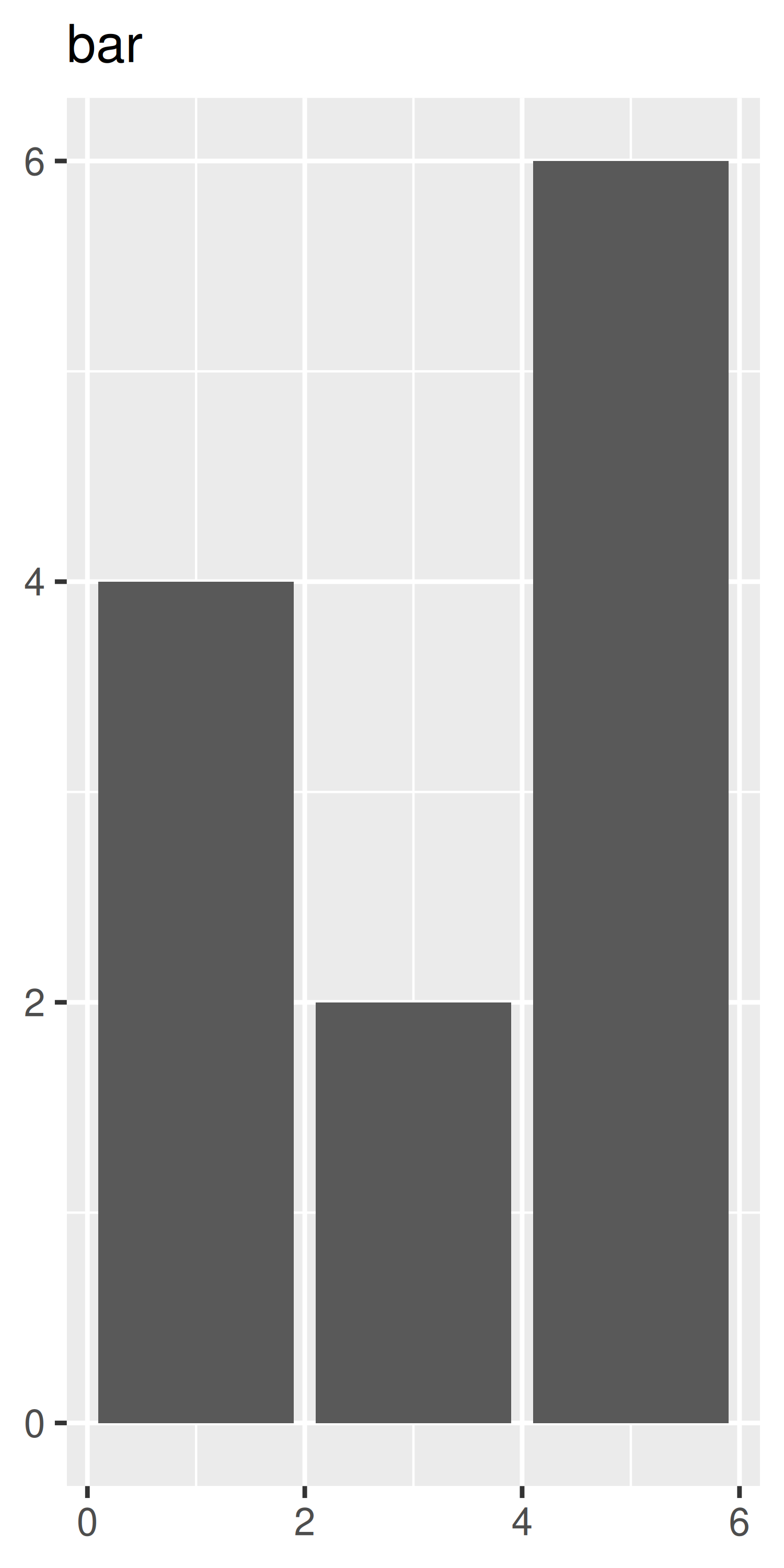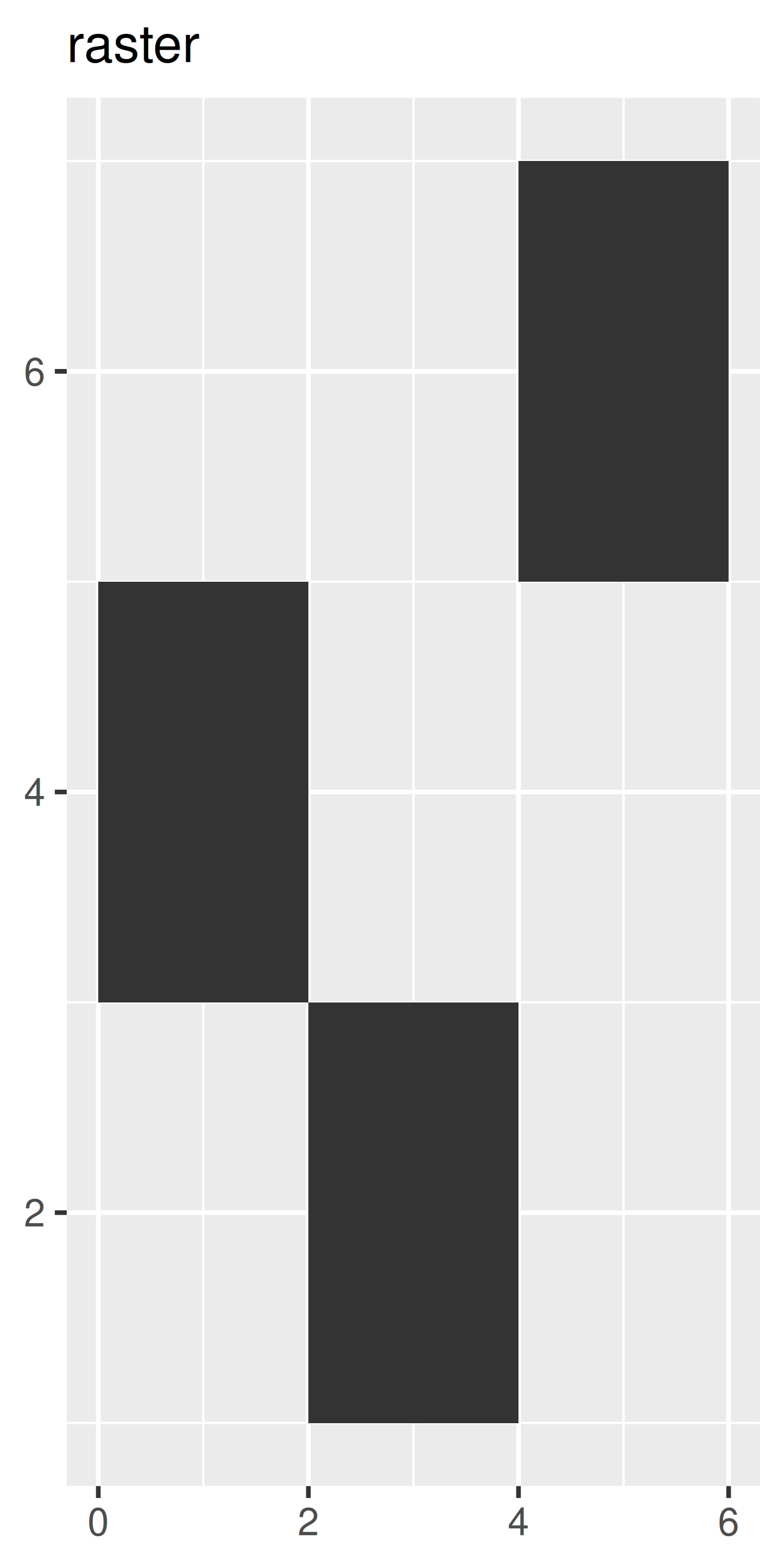p + geom_line() + ggtitle("line")
p + geom_area() + ggtitle("area")
p + geom_path() + ggtitle("path")
p + geom_polygon() + ggtitle("polygon")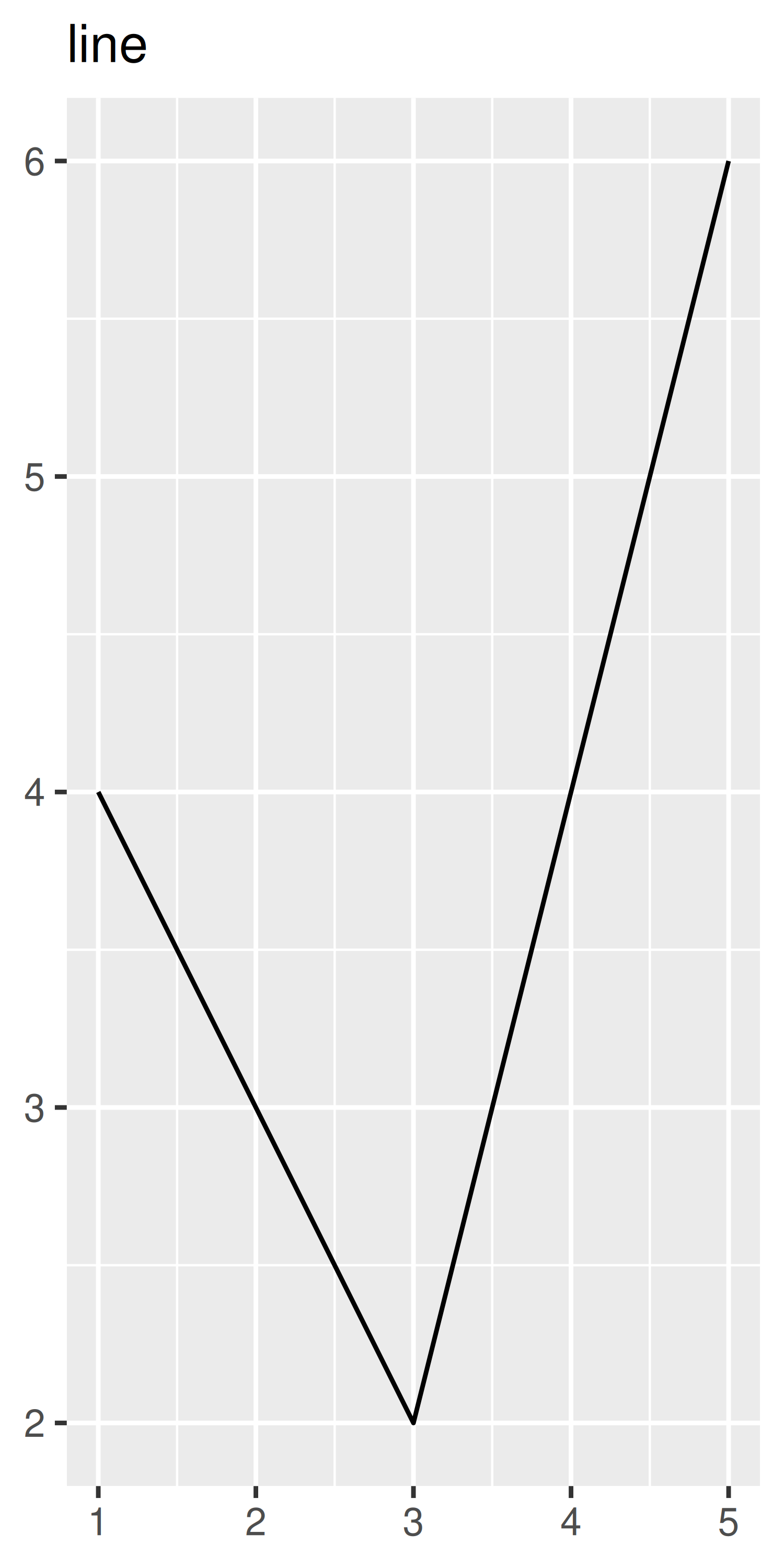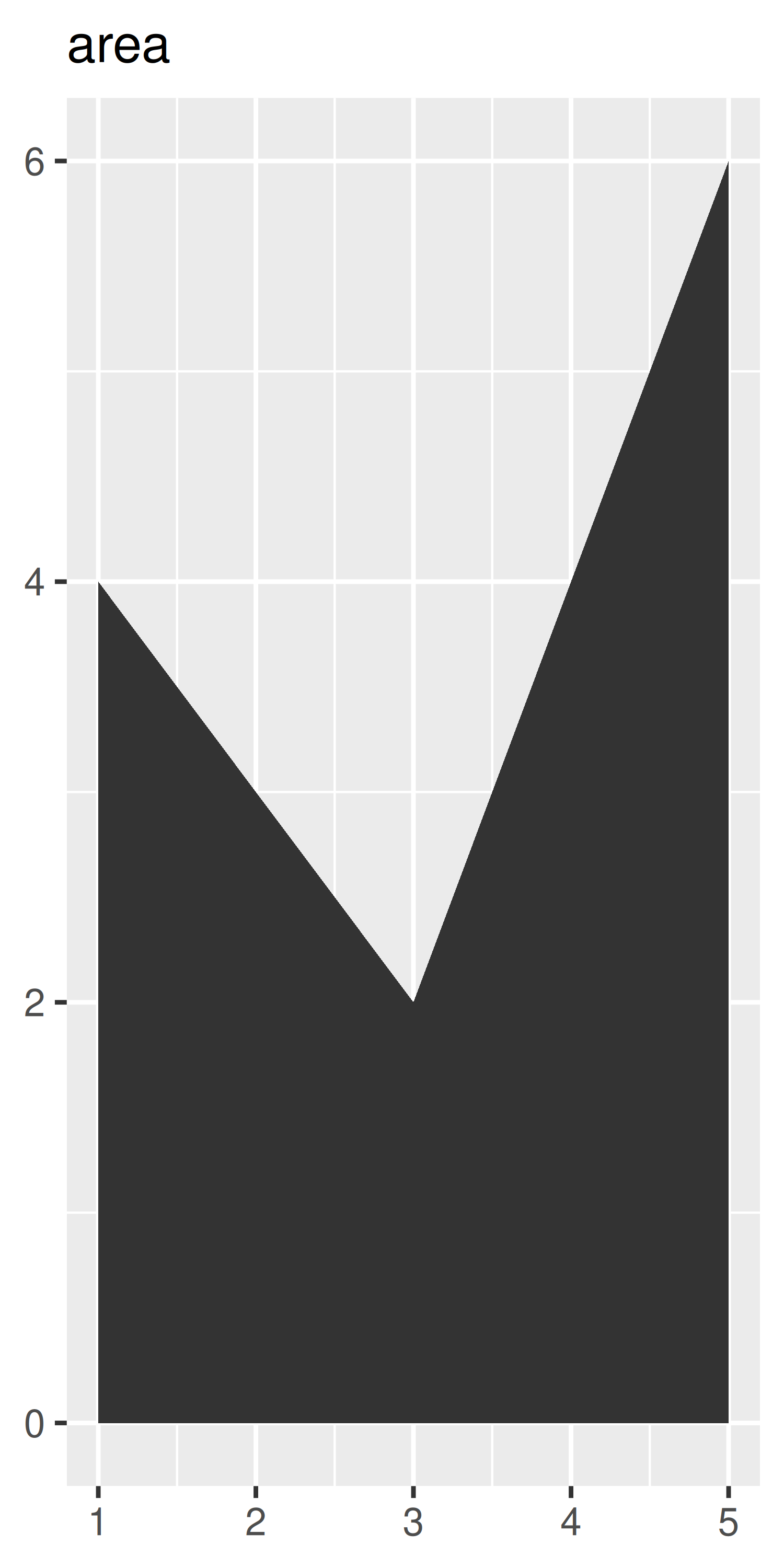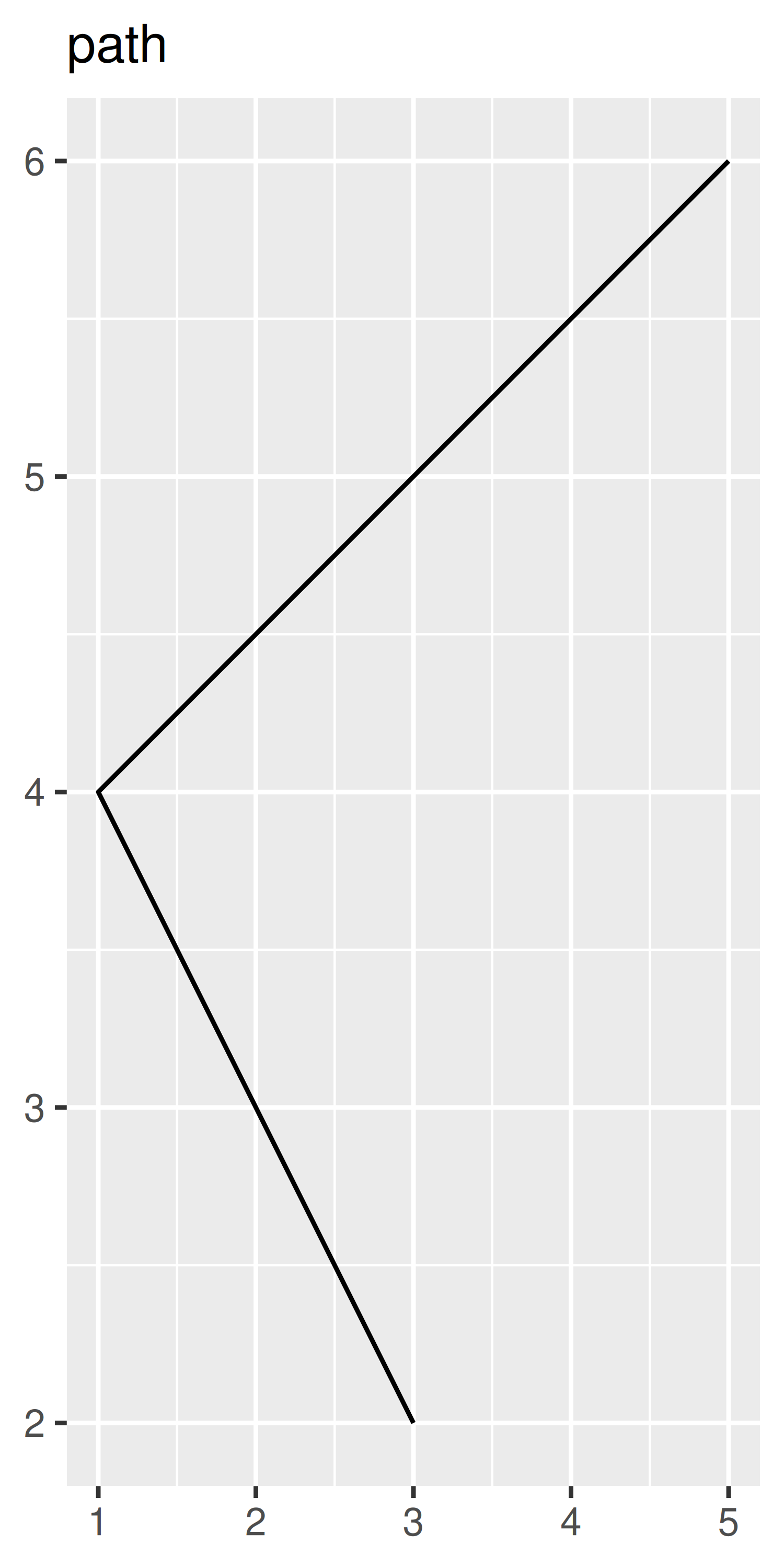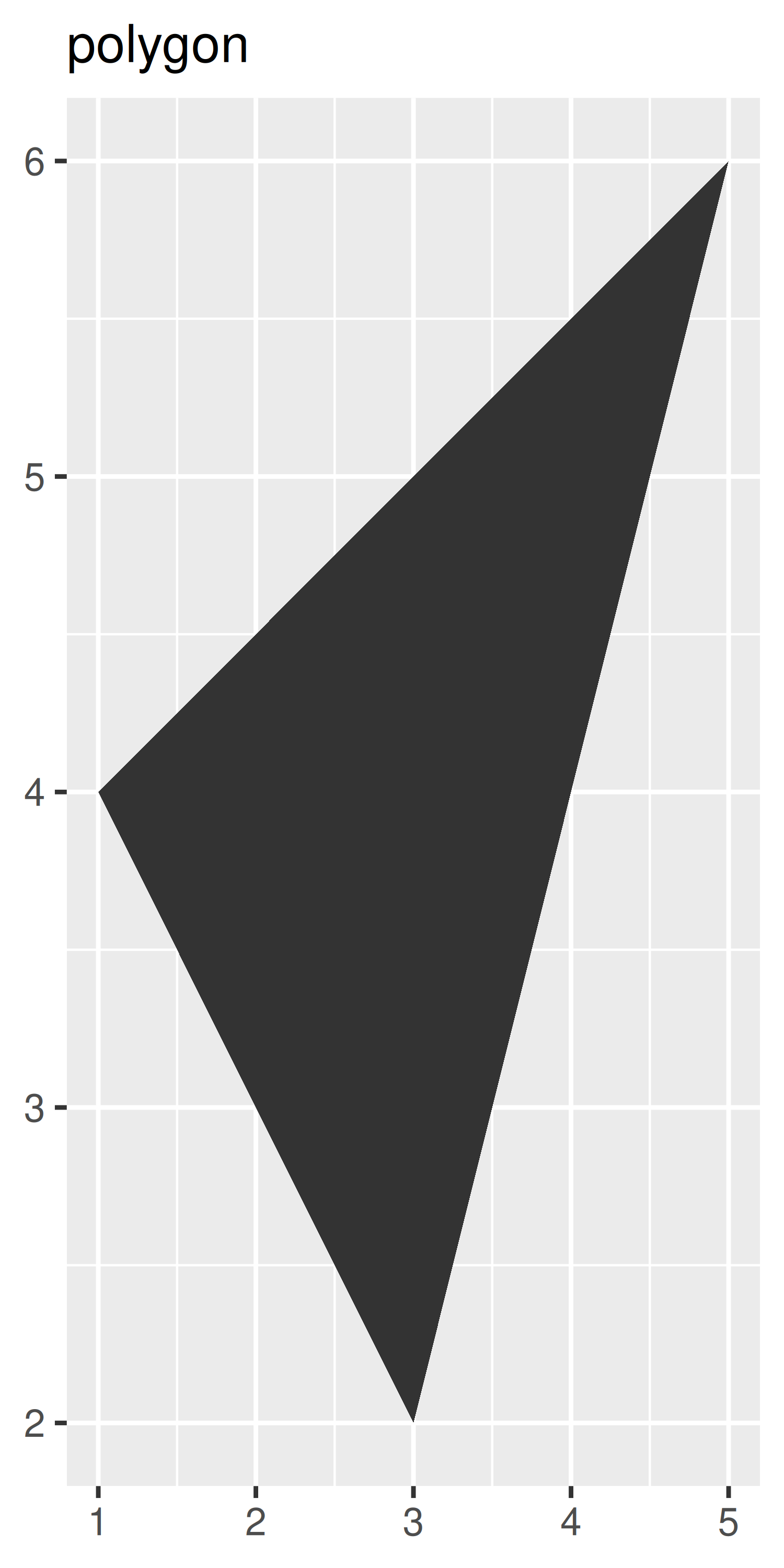3.1.1 Exercises

1. What geoms would you use to draw each of the following named plots?

1. Scatterplot
2. Line chart
3. Histogram
4. Bar chart
5. Pie chart
2. What’s the difference between geom_path() and geom_polygon()? What’s the difference between geom_path() and geom_line()?

3. What low-level geoms are used to draw geom_smooth()? What about geom_boxplot() and geom_violin()?